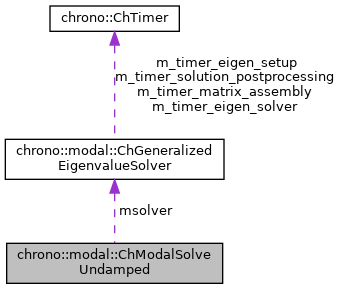chrono::modal::ChModalSolveUndamped Class Reference

## Description

Class for computing eigenvalues/eigenvectors for the undamped constrained system.

It dispatches the settings to some solver of ChGeneralizedEigenvalueSolver class. It handles multiple runs of the solver if one wants to find specific ranges of frequencies. Finally it guarantees that eigenvalues are sorted in the appropriate order of increasing frequency.

#include <ChEigenvalueSolver.h>

Collaboration diagram for chrono::modal::ChModalSolveUndamped:[legend]

## Public Member Functions

ChModalSolveUndamped (int n_lower_modes, double base_freq=1e-5, int max_iters=500, double mtolerance=1e-10, bool mverbose=false, const ChGeneralizedEigenvalueSolver &asolver=ChGeneralizedEigenvalueSolverLanczos())
Constructor for the case of N lower modes. More...

ChModalSolveUndamped (std::vector< ChFreqSpan > mfreq_spans, int max_iters=500, double mtolerance=1e-10, bool mverbose=false, const ChGeneralizedEigenvalueSolver &asolver=ChGeneralizedEigenvalueSolverLanczos())
Constructor for the case of multiple spans of frequency analysis ex. More...

virtual int Solve (const ChSparseMatrix &M, const ChSparseMatrix &K, const ChSparseMatrix &Cq, ChMatrixDynamic< std::complex< double >> &V, ChVectorDynamic< std::complex< double >> &eig, ChVectorDynamic< double > &freq) const
Solve the constrained eigenvalue problem (-wsquare*M + K)*x = 0 s.t. More...

## Public Attributes

std::vector< ChFreqSpan > freq_spans

double tolerance = 1e-10
tolerance for the iterative solver.

int max_iterations = 500
upper limit for the number of iterations. If too low might not converge.

bool verbose = false
turn to true to see some diagnostic.

const ChGeneralizedEigenvalueSolvermsolver

## ◆ ChModalSolveUndamped() [1/2]

 chrono::modal::ChModalSolveUndamped::ChModalSolveUndamped ( int n_lower_modes, double base_freq = 1e-5, int max_iters = 500, double mtolerance = 1e-10, bool mverbose = false, const ChGeneralizedEigenvalueSolver & asolver = ChGeneralizedEigenvalueSolverLanczos() )
inline

Constructor for the case of N lower modes.

Ex. ChModalSolveUndamped(7); finds first 7 lowest modes, using default settings (i.e. the ChGeneralizedEigenvalueSolverLanczos). Ex. ChModalSolveUndamped(5, 1e-5, 500, 1e-10, false, ChGeneralizedEigenvalueSolverKrylovSchur()); finds first 5 lowest modes using the ChGeneralizedEigenvalueSolverKrylovSchur() solver.

Parameters
 n_lower_modes n of lower modes base_freq frequency to whom the nodes are clustered. Use 1e-5 to get n lower modes. As sigma in shift&invert, as: sigma = -pow(base_freq * CH_C_2PI, 2). Too small gives ill conditioning (no convergence). Too large misses rigid body modes. max_iters upper limit for the number of iterations. If too low might not converge. mtolerance tolerance for the iterative solver. mverbose turn to true to see some diagnostic. asolver solver to use (default Lanczos)

## ◆ ChModalSolveUndamped() [2/2]

 chrono::modal::ChModalSolveUndamped::ChModalSolveUndamped ( std::vector< ChFreqSpan > mfreq_spans, int max_iters = 500, double mtolerance = 1e-10, bool mverbose = false, const ChGeneralizedEigenvalueSolver & asolver = ChGeneralizedEigenvalueSolverLanczos() )
inline

Constructor for the case of multiple spans of frequency analysis ex.

ChModalSolveUndamped({{10,1e-5,},{5,40}} , 500) ; finds first 10 lower modes, then 5 modes closest to 40 Hz, etc., using multiple runs of the solver. Closest mean that some could be higher than 40Hz, others can be lower. Another example: suppose you want the 5 lowest modes, then you also are interested in 1 high frequency mode whose frequency is already know approximately, ex. 205 Hz, then you can do ChModalSolveUndamped({{5,1e-5,},{1,205}}, ...). Note about overlapping ranges: if n-th run finds frequencies up to X Hz, and the (n+1)-th run finds some frequency with Y Hz where Y < X, then such Y mode(s) is discarded.

Parameters
 mfreq_spans vector of {nmodes,freq}_i , will provide first nmodes_i starting at freq_i per each i vector entry max_iters upper limit for the number of iterations. If too low might not converge. mtolerance tolerance for the iterative solver. mverbose turn to true to see some diagnostic. asolver solver to use (default Lanczos)

## ◆ Solve()

 int chrono::modal::ChModalSolveUndamped::Solve ( const ChSparseMatrix & M, const ChSparseMatrix & K, const ChSparseMatrix & Cq, ChMatrixDynamic< std::complex< double >> & V, ChVectorDynamic< std::complex< double >> & eig, ChVectorDynamic< double > & freq ) const
virtual

Solve the constrained eigenvalue problem (-wsquare*M + K)*x = 0 s.t.

Cq*x = 0 Return the n. of found modes, where n is not necessarily n_lower_modes (or the sum of ChFreqSpan::nmodes if multiple spans)

Parameters
 M input M matrix, n_v x n_v K input K matrix, n_v x n_v Cq input Cq matrix of constraint jacobians, n_c x n_v V output matrix n x n_v with eigenvectors as columns, will be resized eig output vector with n eigenvalues, will be resized. freq output vector with n frequencies [Hz], as f=w/(2*PI), will be resized.

The documentation for this class was generated from the following files:
• /builds/uwsbel/chrono/src/chrono_modal/ChEigenvalueSolver.h
• /builds/uwsbel/chrono/src/chrono_modal/ChEigenvalueSolver.cpp